Trial ends in
JoVE Science Education
General Chemistry

A subscription to JoVE is required to view this content.
You will only be able to see the first 20 seconds.

Freezing-Point Depression to Determine an Unknown Compound
##### Science Education (General Chemistry)
Determining the Density of a Solid and Liquid
##### Science Education (General Chemistry)
Le Châtelier's Principle

# Freezing-Point Depression to Determine an Unknown Compound

### Overview

Source: Laboratory of Lynne O' Connell — Boston College

When a solid compound is dissolved in a solvent, the freezing point of the resulting solution is lower than that of the pure solvent. This phenomenon is known as freezing-point depression, and the change in temperature is directly related to the molecular weight of the solute. This experiment is designed to find the identity of an unknown compound by using the phenomenon of freezing-point depression to determine its molecular weight. The compound will be dissolved in cyclohexane, and the freezing point of this solution, as well as that of pure cyclohexane, will be measured. The difference between these two temperatures allows for the calculation of the molecular weight of the unknown substance.

### Principles

Certain properties of a solution differ from those of a pure solvent due to interactions that take place between the solute and solvent molecules. The properties that exhibit such changes are called the colligative properties and include vapor-pressure lowering, boiling-point elevation, freezing-point depression, and changes in osmotic pressure. These properties are dependent only upon the number of particles dissolved in the solvent, not on the identity of the particles. A particle, in this instance, is defined as an ion or a molecule. This experiment focuses on the property of freezing-point depression.

When a particular solute is dissolved in a solvent, the following expression holds true:

ΔT = Tf° - Tf = Kfm

The terms Tf° and Tf refer to the freezing-point temperatures of the pure solvent and the solution, respectively. The term "m" indicates the molality of the solution, which is defined as the number of moles of solute per 1,000 g of solvent. This quantity is used, rather than molarity, because it is not temperature dependent. The constant, Kf, is referred to as the freezing-point-depression constantand is dependent only upon the solvent. The change in temperature is also dependent upon the number of solute particles in solution - the more particles present, the larger the change in temperature. For this reason, the previous equation is sometimes written as:

Tf° - Tf = Kfim

where i = the number of solute particles produced per formula unit that dissolves. In a solution containing an electrolyte, each ion is considered to be a particle.

This experiment uses cyclohexane, an organic compound that is a liquid at room temperature, as the solvent. The unknown compound is a non-ionic organic molecule; therefore, i is equal to 1. The molecular weight of this unknown compound can be determined by observing the freezing point of a solution of the compound in cyclohexane and comparing it to the freezing point of pure cyclohexane.

The compound cyclohexane has a melting point (or freezing point) of about 6 °C. A series of temperatures of pure cyclohexane are obtained as it cools down from room temperature through its freezing point in an ice bath. These temperatures are then plotted as a function of time. Similarly, temperatures of a solution of the unknown compound dissolved in cyclohexane are obtained as it cools down to the freezing point, which are also plotted. The plots should look similar to the plots in Figure 1. The Tf° and Tf values can be extrapolated, as shown. In Figure 1b, the temperature does not remain entirely constant as the solution freezes. The freezing point of the solution is the point at which it first begins to freeze and is indicated graphically by a change in the slope of the temperature-time curve.

The molality, m, of a solution can be expressed in terms of the molar mass of the solute: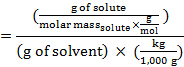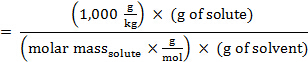Substituting this expression into the equation for freezing-point depression (where i = 1), obtains:Rearranging to solve for molar mass, obtains: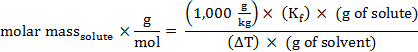The molecular weight (in amu) of a substance has the same numerical value as its molar mass.

The unknown substance is one of the following compounds:

• Biphenyl (C12H10)
• 2-Bromochlorobenzene (C6H4BrCl)
• Naphthalene (C10H8)
• Anthracene (C14H10)
• 1,4-Dibromobenzene (C6H4Br2)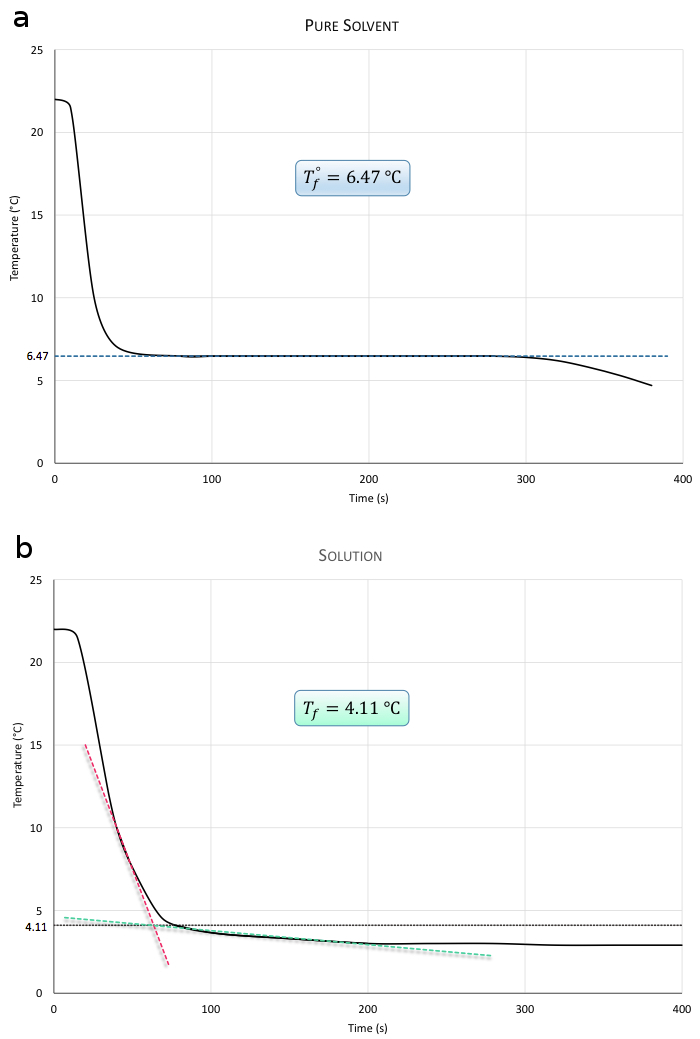Figure 1. Figure 1a is a plot of temperature as a function of time for the determination of Tf° for the pure solvent. Figure 1b is a plot of temperature as a function of time for the determination of Tf for the solution.

### Procedure

A temperature probe interfaced to a computer is used to acquire the temperature readings in this experiment. The temperature probe has an uncertainty of ±0.1 °C.

1. Setting the Parameters in the Software

1. Set the length of the experiment to 800 s.
2. Set the sampling rate to 1 sample per second.
3. Set the upper limit for the temperature range to 40 °C and the lower limit to 0 °C.

2. Measuring the Freezing Point of Cyclohexane

1. Dispense 12.0 mL of cyclohexane from the dispensing bottle into a test tube that is clean and dry.
Caution: Cyclohexane is a flammable solvent.
2. Wipe the temperature probe with a lint-free wipe to be sure it is dry.
3. Insert the stopper with the temperature probe and wire stirrer into the test tube.
4. Make sure the tip of the temperature probe is in the center of the liquid and not touching the sides or bottom of the test tube.
5. Fill a 600-mL beaker about one-third full of water, and add ice until the beaker is three-fourths full.
6. Start the data collection. The computer acquires a temperature reading every second.
7. Move the test tube into the ice-water bath and hold it so the level of liquid in the test tube is below the level of water in the bath.
8. Immediately begin stirring the liquid with the wire stirrer, continuously and at a constant rate.
9. Once freezing begins, as long as liquid and solid are both present, the temperature remains constant until the entire mass has solidified. Allow the computer to continue recording the temperature until the plot has leveled off at a constant temperature.
Note that once the cyclohexane has frozen solid, the temperature starts to decrease again.
10. When a sufficient number of data points have been collected, stop the data collection.
11. Remove the test tube from the ice-water bath and let it warm up to room temperature.
12. Save the data.
13. Adjust the y-axis limits so the plot fills the page. Title the graph, and then print it.

3. Preparing a Solution of the Unknown Compound

1. Accurately weigh 0.14 g of the solid unknown material onto a piece of weighing paper.
2. Check to be sure the cyclohexane contained in the test tube has melted.
3. Remove the stopper from the test tube and carefully add the unknown solid to the cyclohexane, avoiding the loss of any compound adhering to the sides of the test tube or stopper.
4. Replace the stopper and re-weigh the paper to account for any crystals that remain on it.
5. Stir the solution in order to completely dissolve the solid. It is important that no crystals remain.
6. Make a new ice-water bath.

4. Measuring the Freezing Point of the Unknown Compound

1. Prepare the computer to collect a second set of data.
2. Start the data collection.
3. Move the test tube that contains the solution into the ice-water bath.
4. Immediately begin stirring the solution continuously and at a constant rate.
5. Collect the data for 300–500 s in order to clearly see the change in slope that occurs as the solution freezes.
6. Stop the data collection.
7. Save the data, adjust the limits of the y-axis, title the graph, and print it.
8. Do not throw any cyclohexane or unknown compound down the sink. Pour the liquid mixture into the "Laboratory Waste" jar. Rinse the test tube and temperature probe with acetone to remove the last traces of any crystals, pouring the rinses in the waste jar.

Freezing-point depression is the phenomenon that is observed when the freezing point of a solution is lower than that of the pure solvent.

This phenomenon results from interactions between the solute and solvent molecules. The difference in freezing temperatures is directly proportional to the number of solute particles dissolved in the solvent.

The molar mass of a non-volatile solute can be calculated from the difference in freezing temperatures if the masses of the solvent and the solute in the solution are known.

This video will introduce the relationship between freezing-point depression and the molar mass of the solute, a procedure for determining molar mass of an unknown solute, and some real world applications of inducing and observing changes in freezing temperature.

Freezing point depression is a colligative property, meaning it is only affected by the ratio of solute to solvent particles, and not their identity.

At the freezing point of a pure substance, the rates of melting and freezing are equal.

When a solution is cooled to the freezing point of its solvent, the solvent molecules begin to form a solid. It is less energetically favorable to form a mixed lattice of solvent and solute particles. The solute particles remain in the solution phase. Only solvent-solvent interactions contribute to lattice formation, so solvent-solute interactions reduce the rate of freezing compared to that of the pure solvent.

The temperature at which freezing begins is the freezing point of the solution. The solution continues cooling as it freezes, but this continued decrease in temperature reflects the increasing concentration of solute in the solution phase.

Eventually, the solution temperature is so low and so little solvent remains in the liquid phase that it becomes favorable for the solute particles to form a lattice. Once this point is reached, the temperature remains approximately constant until the mixture has frozen solid.

The molar mass of the solute, and therefore the identify of the solute, can be determined from the relationship between the freezing point of the pure solvent, the freezing point of the solution, and the molality of the solution. Molality, or m, is a measure of concentration in moles of the solute per kilogram of the solvent. This relationship depends on the the freezing point depression constant of the solvent and the number of solute particles produced per formula unit that dissolves.

Molality can be expressed in terms of molar mass, so the equation can be rearranged to solve for the molar mass of the solute. Plugging this into the freezing point equation allows the elucidation of the molar mass, once the temperature difference is known. Now that you understand the phenomenon of freezing point depression, let's go through a procedure for determining the molar mass of an unknown solute from freezing point temperatures. The solute is a non-ionic, non-volatile organic molecule that produces one particle per formula unit dissolved, and the solvent is cyclohexane.

To begin this experiment, connect the temperature probe to the computer for data collection. Insert the temperature probe and a stirrer into the sample container.

Set the length of data collection and the rate of sampling. Allow sufficient time in the data collection for the sample to freeze.

Set upper and lower limits of the temperature range to sample.

Add 12 mL of cyclohexane to a clean, dry test tube. Wipe the temperature probe with a Kimwipe. Insert the stopper assembly into the test tube such that the tip of the temperature probe is centered in the liquid and does not touch the sides or bottom.

In a beaker, prepare an ice water bath. Then, start the temperature data collection.

Place the test tube into the ice water bath, ensuring that the level of liquid in the test tube is below the surface. Continuously stir the liquid at a constant rate.

Once freezing begins, allow data collection to continue until the plot has leveled off at a constant temperature. This is the freezing point of pure cyclohexane. Remove the test tube from the ice water bath and allow it to warm to room temperature.

Once the cyclohexane has melted, accurately weigh the solid unknown material on weighing paper. Remove the stopper from the test tube and add the solid. Avoid allowing compound to adhere to the test tube.

Replace the stopper and stir the solution until the solid is completely dissolved. It is important that no solid crystals remain.

Set the parameters for data collection and prepare a fresh ice water bath. Start collection, place the test tube into the bath, and stir continuously at a constant rate. Once freezing begins, the freezing point continues to decrease due to the increasing solute concentration. Continue collecting data until the slope of this decrease is evident. When the experiment has finished, allow the solution of the unknown compound to warm to room temperature and then dispose of it according to the procedures for organic waste.

In this experiment, the unknown substance is known to be one of five possible compounds: biphenyl, bromochlorobenzene, naphthalene, anthracene, and dibromobenzene. The identity of the unknown can be determined by comparing its molar mass to these known substances.

The unknown solute produces one particle per formula unit dissolved. To calculate the molar mass of the unknown compound, the freezing point depression constant of cyclohexane, the mass of solute and solvent used, and the difference in freezing temperatures are all needed.

0.147 g of the unknown solute were used in this example. The freezing point depression constant of cyclohexane is 20.2 °C-kg per mol of solute. The density and volume of cyclohexane are used to calculate the mass of the solvent.

The values of the freezing point of the pure solvent and the freezing point of the solution are determined from the plots.

If the compound is known to be one of a few possible compounds, as in this experiment, the molar mass can simply be compared to those compounds. Of the five options provided for this experiment, naphthalene is the closest match.

The phenomenon of freezing point depression has many applications both inside and outside the laboratory.

Calcium chloride is preferred to sodium chloride for treating icy roads because of the effects of freezing point depression. As calcium chloride releases one more particle than sodium chloride does, it depresses the freezing point of water further and thus melts ice at lower temperatures.

In this study, a melting experiment was conducted with two different iron-sulfur mixtures. The sample with the higher mass fraction of sulfur was completely liquid at the temperature of the experiment, whereas the sample with less sulfur was still partially solid. This demonstrates that with increased impurities, in this case sulfur, the observed melting point is lower than for the pure solid. Here, the melting point differences between the two samples lend insight into the formation of the Earth's core.

You've just watched JoVE's introduction to using freezing point depression to determine the identity of an unknown compound. You should now understand the phenomenon of freezing point depression, the relationship between freezing point depression and the molar mass of the solute, and why the phenomenon is useful to a variety of industries.

Thanks for watching!

### Results

The mass of cyclohexane that was dispensed can be calculated. The density of cyclohexane is 0.779 g/mL.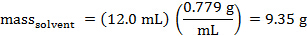The values for Tf° and Tf can be determined from the plots.

The molar mass, and thus the molecular weight, of the unknown compound can also be calculated. For cyclohexane, Kf = 20.2 °C kg/mole of solute.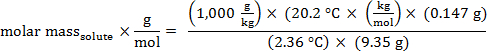molar mass = 134 g/mol

molecular weight = 134 amu

The molecular weights of the possible compounds are:

• 154.21 amu for Biphenyl
• 191.46 amu for 2-Bromochlorobenzene
• 128.17 amu for Naphthalene
• 178.23 amu for Anthracene
• 235.90 amu for 1,4-Dibromobenzene

The value determined experimentally for the molecular weight of the unknown compound is closest to the literature value for naphthalene.

The percent error can be calculated.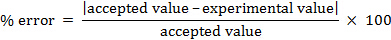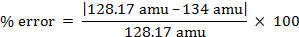% error = 4.55%

### Applications and Summary

Perhaps the most visible application of the phenomenon of freezing-point depression occurs during the winter months, when roads and sidewalks become icy, and salt is used to treat the slippery surfaces. When the salt mixes with the ice, the freezing point of the water is depressed so the ice melts at a lower temperature. Because the degree of the freezing point depression is dependent on the number of particles in solution, salts that release three ions per formula unit, such as calcium chloride (CaCl2), are often utilized for this purpose. Ice cream makers also make use of the freezing-point depression that occurs when salt and ice are mixed. The freezing point of cream is well below 0 °C, especially when it is combined with sugar and other ingredients used to make ice cream. For this reason, ice and rock salt are combined in the outer container of an ice cream maker in order to achieve a temperature low enough to freeze the mixture enclosed in the inner container.

Chemists exploit the phenomenon of freezing-point depression in the analysis of solid organic compounds. The purity of a solid product from a chemical synthesis is often determined by measuring the melting point (theoretically, the same as the freezing point) of the material. If an impurity is present in the compound, the observed melting point is lower than expected. This occurs because, as the solid starts to melt, the impurity acts as a solute that is dissolved in the liquid form of the compound; thus, the melting, or freezing, point of the compound is depressed.

The pharmaceutical industry uses large amounts of organic solvents for reactions that lead to the synthesis of therapeutic agents. These solvents create substantial volumes of liquid waste that are hazardous to the environment. Occasionally, it is possible to take advantage of the freezing-point depression phenomenon to eliminate the need for a solvent in a synthesis. When solid reactants involved in a reaction are crushed together, the melting (or freezing) points of the two compounds are lowered. If the two compounds each have a very low melting point, the pair actually become liquids at room temperature when they are ground together, which allows the molecules to interact with each other so the reaction can occur. These solvent-free processes are an example of "green" chemistry, which refers to chemical procedures that reduce or eliminate the use and generation of hazardous substances.X